### Da Silva Method (Variance-Component Moving Average Model)

The Da Silva method assumes that the observed value of the dependent variable at the tth time point on the ith cross-sectional unit can be expressed as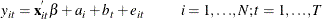where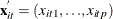is a vector of explanatory variables for the tth time point and ith cross-sectional unit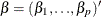is the vector of parameters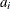is a time-invariant, cross-sectional unit effect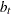is a cross-sectionally invariant time effect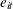is a residual effect unaccounted for by the explanatory variables and the specific time and cross-sectional unit effects

Since the observations are arranged first by cross sections, then by time periods within cross sections, these equations can be written in matrix notation as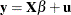where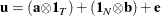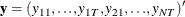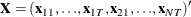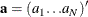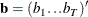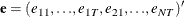Here 1is an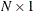vector with all elements equal to 1, and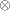denotes the Kronecker product.

The following conditions are assumed:

1.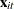is a sequence of nonstochastic, known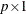vectors in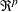whose elements are uniformly bounded in. The matrix X has a full column rank p.

2.is a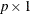constant vector of unknown parameters.

3. a is a vector of uncorrelated random variables such that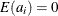and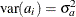,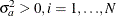.

4. b is a vector of uncorrelated random variables such that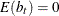and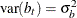where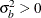and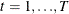.

5.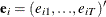is a sample of a realization of a finite moving-average time series of order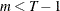for each i ; hence,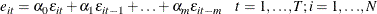where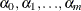are unknown constants such that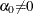and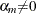, and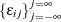is a white noise process for each—that is, a sequence of uncorrelated random variables with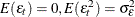, and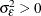.for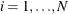are mutually uncorrelated.

6. The sets of random variables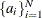,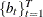, and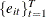forare mutually uncorrelated.

7. The random terms have normal distributions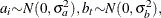and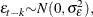for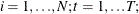and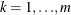.

If assumptions 1–6 are satisfied, then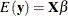and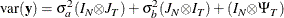where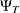is a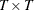matrix with elements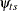as follows: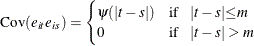where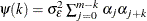for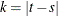. For the definition of
,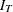,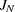, and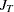, see the section Fuller and Battese’s Method.

The covariance matrix, denoted by V, can be written in the form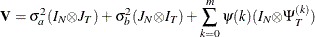where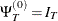, and, for k =1,, m,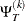is a band matrix whose kth off-diagonal elements are 1’s and all other elements are 0’s.

Thus, the covariance matrix of the vector of observations y has the form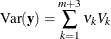where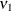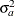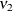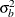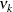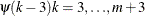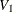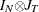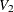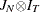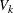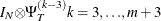The estimator ofis a two-step GLS-type estimator—that is, GLS with the unknown covariance matrix replaced by a suitable estimator of V. It is obtained by substituting Seely estimates for the scalar multiples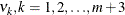.

Seely (1969) presents a general theory of unbiased estimation when the choice of estimators is restricted to finite dimensional vector spaces, with a special emphasis on quadratic estimation of functions of the form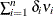.

The parameters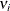(i =1,, n) are associated with a linear model E(y )=Xwith covariance matrix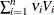where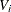(i =1,, n) are real symmetric matrices. The method is also discussed by Seely (1970a,1970b) and Seely and Zyskind (1971). Seely and Soong (1971) consider the MINQUE principle, using an approach along the lines of Seely (1969).